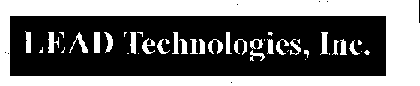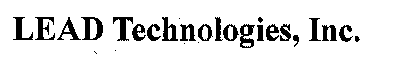﻿ L_InvertedTextBitmap | Function References | Raster Imaging C API Help
In This Topic ▼

# L_InvertedTextBitmap

#include "l_bitmap.h"

L_LTIMGCOR_API L_INT L_InvertedTextBitmap(pBitmap, pInvertedText, pfnCallback, pUserData, uFlags)

Finds and modifies areas of inverted text in a 1-bit black and white image.

## Parameters

### pBITMAPHANDLE pBitmap

Pointer to the bitmap handle referencing the bitmap in which to find inverted text.

### pINVERTEDTEXT pInvertedText

Pointer to the INVERTEDTEXT structure that LEADTOOLS uses to find and correct inverted text.

### INVERTEDTEXTCALLBACK pfnCallback

Optional callback function for additional processing.

If you do not provide a callback function, use NULL as the value of this parameter. If you do provide a callback function, use the function pointer as the value of this parameter.

The callback function must adhere to the function prototype described in INVERTEDTEXTCALLBACK Function.

### L_VOID *pUserData

Void pointer that you can use to pass one or more additional parameters that the callback function needs.

To use this feature, assign a value to a variable or create a structure that contains as many fields as you need. Then, in this parameter, pass the address of the variable or structure, casting it to L_VOID  *. The callback function, which receives the address in its own pUserData parameter, can cast it to a pointer of the appropriate data type to access your variable or structure.

If the additional parameters are not needed, you can pass NULL in this parameter.

### L_UINT32 uFlags

Reserved for future use. Must be 0.

#### Returns

Value Meaning
SUCCESS The function was successful.
< 1 An error occurred. Refer to Return Codes.

This function does not support signed data images. It returns the error code ERROR_SIGNED_DATA_NOT_SUPPORTED if a signed data image is passed to this function.

(Document) This function finds and modifies areas of inverted text that are common in scanned text documents. Below is an example of inverted text:Modifying the area of inverted text yields the following:The behavior of this function can be modified by using its callback.

This function works only on 1-bit black and white images.

If a region is selected, only the selected region will be changed by this function. If no region is selected, the whole image will be processed.

This function does not support 32-bit grayscale images. It returns the error code ERROR_GRAY32_UNSUPPORTED if a 32-bit grayscale image is passed to this function.

Required DLLs and Libraries

Platforms

Win32, x64, Linux.

## Example

This example finds all inverted text regions greater than 5 inches in width and 1/2 inch in height and inverts the text so that it appears normal. The callback is used to display additional information about the inverted text regions. The callback does NOT return a region. A LEAD region is returned by the function showing all of the changes.

````L_INT EXT_CALLBACK InvertedTextCB(HRGN hRgn, PRECT pBoundingRect, L_INT32 iWhiteCount, L_INT32 iBlackCount, L_VOID* pUserData) `
`{ `
`   UNREFERENCED_PARAMETER(pUserData); `
` `
`   L_TCHAR  szMsg; `
` `
`   //Note: no hRgn to delete because it was not requested `
`   RECT rcRect; `
` `
`   GetRgnBox(hRgn, &rcRect); `
`   wsprintf( `
`      szMsg,  `
`      TEXT("Region Bounds: left=%d, right=%d, top=%d, bottom=%d \n"), `
`      rcRect.left, rcRect.right, rcRect.top, rcRect.bottom); `
`   OutputDebugString(szMsg); `
`   DeleteObject(hRgn); `
` `
`   wsprintf(szMsg, `
`            TEXT("Size[%dx%d]\tBounds[%d,%d][%d,%d]\tWhiteCount[%d]\tBlackCount[%d]\n"), `
`            pBoundingRect->left, `
`            pBoundingRect->top, `
`            pBoundingRect->right, `
`            pBoundingRect->bottom, `
`            iWhiteCount, `
`            iBlackCount); `
`   OutputDebugString(szMsg); `
`   return SUCCESS_REMOVE; `
`} `
` `
`L_INT InvertedTextBitmapExample(pBITMAPHANDLE pBitmap) `
`{ `
`   L_INT32        nRet; `
`   BITMAPHANDLE   BitmapRegion; `
`   INVERTEDTEXT   it; `
`    `
`   memset(&BitmapRegion, 0, sizeof(BITMAPHANDLE)); `
` `
`   it.uStructSize = sizeof(INVERTEDTEXT); `
` `
`   //Units are in thousandths of an inch `
`   it.iMinInvertWidth   = 6000; `
`   it.iMinInvertHeight  = 375; `
`   it.iMinBlackPercent  = 75; `
`   it.iMaxBlackPercent  = 95; `
`   it.pBitmapRegion     = &BitmapRegion; `
`   it.uBitmapStructSize = sizeof(BITMAPHANDLE); `
`   it.uFlags            = INVERTEDTEXT_SINGLE_REGION | INVERTEDTEXT_LEAD_REGION | INVERTEDTEXT_USE_DPI | INVERTEDTEXT_CALLBACK_REGION; `
`       `
`   nRet = L_InvertedTextBitmap(pBitmap, `
`                               &it, `
`                               InvertedTextCB, `
`                               NULL, 0); `
`    `
`   if (nRet == SUCCESS) `
`   { `
`      RECT rectRgn; `
`       `
`      //Delete the existing region `
`      L_FreeBitmapRgn(pBitmap); `
`       `
`      nRet = L_GetBitmapRgnBounds(it.pBitmapRegion, NULL, &rectRgn); `
`      if(nRet !=SUCCESS) `
`         return nRet; `
`      if (!IsRectEmpty(&rectRgn)) `
`      { `
`         nRet = L_CopyBitmapHandle(pBitmap, it.pBitmapRegion, sizeof(BITMAPHANDLE)); `
`         if(nRet !=SUCCESS) `
`            return nRet; `
`      } `
`   } `
`   else `
`   { `
`      return nRet; `
`   } `
` `
`   return SUCCESS; `
`} ````

Help Version 20.0.2020.2.18